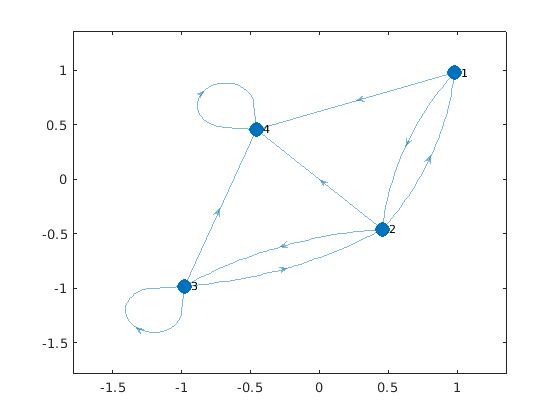Question #6733

# How to visualize a directed graph from its adjacency matrix on Matlab?

The ﻿`digraph()` Matlab function creates a directed graphs object (directional edges connecting the nodes). The following example plot a directed graph from the adjacency matrix :

``````% Adjacency matrix
A = [ 0 1 0 1 ;
1 0 1 1 ;
0 1 1 1 ;
0 0 0 1 ];

% Create directed graph object
G = digraph(A);

% Plot directed graph
p=plot(G, 'MarkerSize', 10);``````

The above code displays the following figure:9 events in history

Icons proudly provided by Friconix.